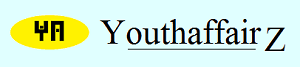May 2013

# Solution

Everyone shook hands with everyone else exactly once and there were 1225 handshakes in all.

Let us suppose that there is only one person, P1, in the hall. He will not have anyone to shake hands with and there will be no handshakes. So the number of handshakes is zero.

Suppose there are two persons in the hall P1 and P2. They will shake hands with each other and the number of handshake will be one.

If there are three persons P1, P2 and P3, then the handshakes will take place between the following pairs: (P1, P2), (P1, P3), (P2, P3). Therefore there will be three handshakes.

If there are four persons P1, P2, P3 and P4 then the handshakes will be between the following pairs: (P1, P2), (P1, P3), (P1, P4), (P2, P3), (P2, P4), (P3, P4). Therefore there will be six handshakes.

If there are five persons P1, P2, P3, P4 and P5 then there will be handshakes between the following pairs: (P1,P2), (P1, P3), (P1, P4), (P1, P5), (P2, P3), (P2, P4), (P2, P5), (P3, P4), (P3, P5), (P4, P5). Therefore there will be ten handshakes.

Likewise, if there are six persons, the number of handshakes will be 15, if there are seven persons the number of handshakes will be 21, and so on.

I can notice a pattern here. If there are "n" number of persons, there will be n (n-1)/2 number of handshakes.

There were 1225 handshakes in the present case. Therefore n (n-1)/2 = 1225

Thus, n (n-1) = 2450

n2 - n - 2450 = 0.

So, n2 - 50n + 49n - 2450 = 0.

Therefore, n (n - 50) + 49 (n - 50) = 0

Therefore, (n - 50) (n + 49) = 0

So, n = 50 or n = -49. But "n" cannot be negative here. Therefore n = 50 and the number of persons in the hall was 50.

Of course, the more sophisticated way of solving this problem is by using the permutation and combination formula. For that, you have to know about permutations and combinations. Suppose there are "n" number of persons; two persons are needed for a handshake. Two persons can be chosen out of "n" number of people in nC2 ways; thus, there will be nC2 handshakes (without repetition).

Now, nC2 = n!/ [(n-2)! x 2!] = 1225. Thus, n(n-1)(n-2)!/ [(n-2)! x 2!] = 1225.

Thus, n(n-1)/2 = 1225. Therefore, n2 - n - 2450 = 0. All that you have to do now is to solve the equation as above.

• Union Public Service Commission - www.upsc.gov.in
• IIT-Kharagpur - www.iitkgp.ac.in
• Indian Statistical Institute - www.isical.ac.in
• Indian Institute of Technology Madras - www.iitm.ac.in
• Indian Institute of Management, Ahmedabad - www.iimahd.ernet.in
• Indian Institute of Mass Commission - www.iimc.nic.in
• IIT Bombay - www.iitb.ac.in